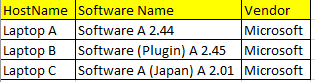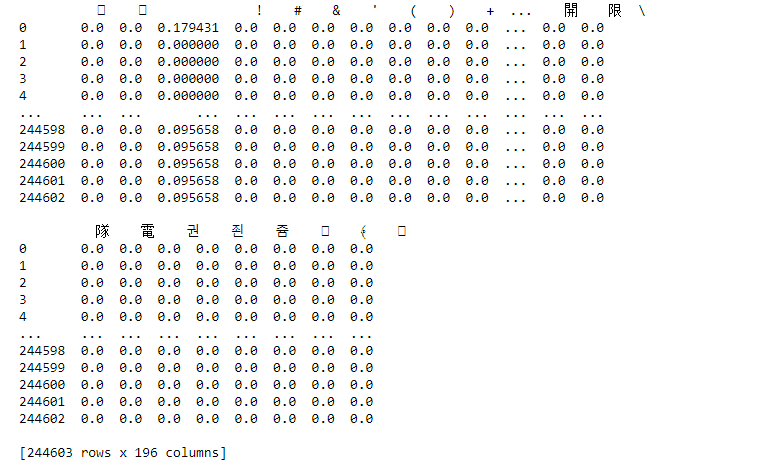Home » Machine Learning in Cyber Security — Malicious Software Installation

# Machine Learning in Cyber Security — Malicious Software Installation

## IntroductionAn example of how the dataset looks like — the 3 entries listed above are referring to the same software

```import pandas as pd
from sklearn.feature_extraction.text import TfidfVectorizer# Import the dataset
field_extracted = df['softwarename']# initialize the TF-IDF
vectorizer = TfidfVectorizer(analyzer='char')
vectors = vectorizer.fit_transform(field_extracted)
feature_names = vectorizer.get_feature_names()
dense = vectors.todense()
denselist = dense.tolist()
result = pd.DataFrame(denselist, columns=feature_names)```The result from the TF-IDF scripts above (with a mix of different languages e.g. Korean, Chinese)
```import math
from collections import Counter# Function of calculating Entropy
def eta(data, unit='natural'):
base = {
'shannon' : 2.,
'natural' : math.exp(1),
'hartley' : 10.
}if len(data) <= 1:
return 0counts = Counter()for d in data:
counts[d] += 1ent = 0probs = [float(c) / len(data) for c in counts.values()]
for p in probs:
if p > 0.:
ent -= p * math.log(p, base[unit])return ententropy  = [eta(x) for x in field_extracted]```

You can also read this article on our Mobile APP